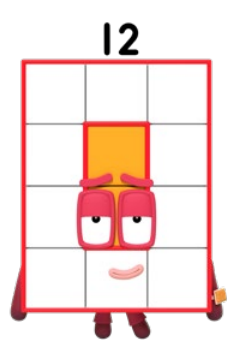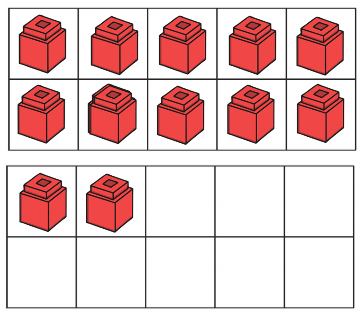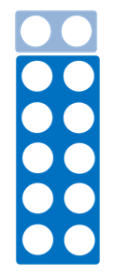# 6. Twelve

We watched the Numberblocks 'Twelve' episode...We learnt that number 12 is a 1 and a 2, and that the first 1 is actually a 10!

We learnt that the number 12 is what we call a ‘teen number’. The teen numbers start with a 1. They are made up of 10 and a bit more. In the Numberblocks episode we saw that 10 and 2 make 12.

We practised representing number 12...

- We realised that we can't make number 12 on our fingers so worked with a friend to show 12.

- We practised writing the number 12 in the air, on the carpet and on whiteboards.

- We used tens frames. We know that when we have a full ten frame that there are 10 ones. We can say ‘ten ones is equal to one ten!’ We know now that one ten and a two means there is 12!- We also made 12 using Numicon pieces and found number 12 on a number line, looking carefully at which numbers come before and after 12.Finally we practised making 12 with resources. We counted out 12 objects. We made a group of 10 and found we had 2 left. 12 is one 10 and 2, 10 and 2 make 12.